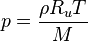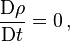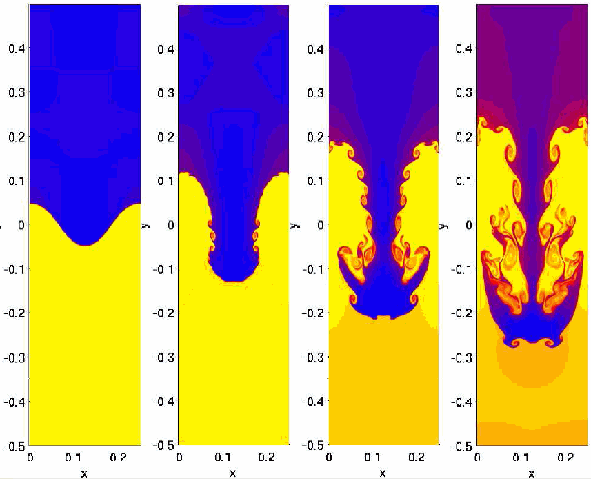Fluid dynamicsBy Wikipedia,
the free encyclopedia,

https://en.wikipedia.org/wiki/Fluid_dynamics

 Continuum mechanicsNavier–Stokes equationsTypical aerodynamic teardrop shape, showing the pressure distribution as the thickness of the black line and showing the velocity in the boundary layer as the violet triangles. The green vortex generators prompt the transition to turbulent flow and prevent back-flow also called flow separation from the high pressure region in the back. The surface in front is as smooth as possible or even employs shark like skin, as any turbulence here will reduce the energy of the airflow. The Kammback also prevents back flow from the high pressure region in the back across the spoilers to the convergent part. Putting stuff inside out results in tubes; they also face the problem of flow separation in their divergent parts, so called diffusers. Cutting the shape into halves results in an aerofoil with the low pressure region on top leading to lift (force).

In physics, fluid dynamics is a sub-discipline of fluid mechanics that deals with fluid flow — the natural science of fluids (liquids and gases) in motion. It has several subdisciplines itself, including aerodynamics (the study of gases in motion) and hydrodynamics (the study of liquids in motion). Fluid dynamics has a wide range of applications, including calculating forces and moments on aircraft, determining the mass flow rate of petroleum through pipelines, predicting weather patterns, understanding nebulae in interstellar space and reportedly modeling fission weapon detonation. Some of its principles are even used in traffic engineering, where traffic is treated as a continuous fluid.

Fluid dynamics offers a systematic structure that underlies these practical disciplines, that embraces empirical and semi-empirical laws derived from flow measurement and used to solve practical problems. The solution to a fluid dynamics problem typically involves calculating various properties of the fluid, such as velocity, pressure, density, and temperature, as functions of space and time.

Historically, hydrodynamics meant something different than it does today. Before the twentieth century, hydrodynamics was synonymous with fluid dynamics. This is still reflected in names of some fluid dynamics topics, like magnetohydrodynamics and hydrodynamic stability — both also applicable in, as well as being applied to, gases.

## Equations of fluid dynamics

The foundational axioms of fluid dynamics are the conservation laws, specifically, conservation of mass, conservation of linear momentum (also known as Newton's Second Law of Motion), and conservation of energy (also known as First Law of Thermodynamics). These are based on classical mechanics and are modified in quantum mechanics and general relativity. They are expressed using the Reynolds Transport Theorem.

In addition to the above, fluids are assumed to obey the continuum assumption. Fluids are composed of molecules that collide with one another and solid objects. However, the continuum assumption considers fluids to be continuous, rather than discrete. Consequently, properties such as density, pressure, temperature, and velocity are taken to be well-defined at infinitesimally small points, and are assumed to vary continuously from one point to another. The fact that the fluid is made up of discrete molecules is ignored.

For fluids which are sufficiently dense to be a continuum, do not contain ionized species, and have velocities small in relation to the speed of light, the momentum equations for Newtonian fluids are the Navier-Stokes equations, which is a non-linear set of differential equations that describes the flow of a fluid whose stress depends linearly on velocity gradients and pressure. The unsimplified equations do not have a general closed-form solution, so they are primarily of use in Computational Fluid Dynamics. The equations can be simplified in a number of ways, all of which make them easier to solve. Some of them allow appropriate fluid dynamics problems to be solved in closed form.

In addition to the mass, momentum, and energy conservation equations, a thermodynamical equation of state giving the pressure as a function of other thermodynamic variables for the fluid is required to completely specify the problem. An example of this would be the perfect gas equation of state:$p= \frac{\rho R_u T}{M}$

where p is pressure, ρ is density, Ru is the gas constant, M is the molar mass and T is temperature.

### Compressible vs incompressible flow

All fluids are compressible to some extent, that is changes in pressure or temperature will result in changes in density. However, in many situations the changes in pressure and temperature are sufficiently small that the changes in density are negligible. In this case the flow can be modeled as an incompressible flow. Otherwise the more general compressible flow equations must be used.

Mathematically, incompressibility is expressed by saying that the density ρ of a fluid parcel does not change as it moves in the flow field, i.e.,$\frac{\mathrm{D} \rho}{\mathrm{D}t} = 0 \, ,$

where D / Dt is the substantial derivative, which is the sum of local and convective derivatives. This additional constraint simplifies the governing equations, especially in the case when the fluid has a uniform density.

For flow of gases, to determine whether to use compressible or incompressible fluid dynamics, the Mach number of the flow is to be evaluated. As a rough guide, compressible effects can be ignored at Mach numbers below approximately 0.3. For liquids, whether the incompressible assumption is valid depends on the fluid properties (specifically the critical pressure and temperature of the fluid) and the flow conditions (how close to the critical pressure the actual flow pressure becomes). Acoustic problems always require allowing compressibility, since sound waves are compression waves involving changes in pressure and density of the medium through which they propagate.

### Viscous vs inviscid flow

Viscous problems are those in which fluid friction has significant effects on the fluid motion.

The Reynolds number can be used to evaluate whether viscous or inviscid equations are appropriate to the problem.

Stokes flow is flow at very low Reynolds numbers, such that inertial forces can be neglected compared to viscous forces.

On the contrary, high Reynolds numbers indicate that the inertial forces are more significant than the viscous (friction) forces. Therefore, we may assume the flow to be an inviscid flow, an approximation in which we neglect viscosity at all, compared to inertial terms.

This idea can work fairly well when the Reynolds number is high. However, certain problems such as those involving solid boundaries, may require that the viscosity be included. Viscosity often cannot be neglected near solid boundaries because the no-slip condition can generate a thin region of large strain rate (known as Boundary layer) which enhances the effect of even a small amount of viscosity, and thus generating vorticity. Therefore, to calculate net forces on bodies (such as wings) we should use viscous flow equations. As illustrated by d'Alembert's paradox, a body in an inviscid fluid will experience no drag force. The standard equations of inviscid flow are the Euler equations. Another often used model, especially in computational fluid dynamics, is to use the Euler equations away from the body and the boundary layer equations, which incorporates viscosity, in a region close to the body.

The Euler equations can be integrated along a streamline to get Bernoulli's equation. When the flow is everywhere irrotational and inviscid, Bernoulli's equation can be used throughout the flow field. Such flows are called potential flows.

### Steady vs unsteady flowHydrodynamics simulation of the Rayleigh–Taylor instability

When all the time derivatives of a flow field vanish, the flow is considered to be a steady flow. Otherwise, it is called unsteady. Whether a particular flow is steady or unsteady, can depend on the chosen frame of reference. For instance, laminar flow over a sphere is steady in the frame of reference that is stationary with respect to the sphere. In a frame of reference that is stationary than the governing equations of the same problem without taking advantage of the steadiness of the flow field.

Although strictly unsteady flows, time-periodic problems can often be solved by the same techniques as steady flows. For this reason, they can be considered to be somewhere between steady and unsteady.

### Laminar versus turbulent flow

Turbulence is flow characterized by recirculation, eddies, and apparent randomness. Flow in which turbulence is not exhibited is called laminar. It should be noted, however, that the presence of eddies or recirculation alone does not necessarily indicate turbulent flow—these phenomena may be present in laminar flow as well. Mathematically, turbulent flow is often represented via a Reynolds decomposition, in which the flow is broken down into the sum of an average component and a perturbation component.

It is believed that turbulent flows can be described well through the use of the Navier–Stokes equations. Direct numerical simulation (DNS), based on the Navier–Stokes equations, makes it possible to simulate turbulent flows at moderate Reynolds numbers. Restrictions depend on the power of the computer used and the efficiency of the solution algorithm. The results of DNS agree with the experimental data.

Most flows of interest have Reynolds numbers much too high for DNS to be a viable option, given the state of computational power for the next few decades. Any flight vehicle large enough to carry a human (L > 3 m), moving faster than 72 km/h (20 m/s) is well beyond the limit of DNS simulation (Re = 4 million). Transport aircraft wings (such as on an Airbus A300 or Boeing 747) have Reynolds numbers of 40 million (based on the wing chord). In order to solve these real-life flow problems, turbulence models will be a necessity for the foreseeable future. Reynolds-averaged Navier–Stokes equations (RANS) combined with turbulence modeling provides a model of the effects of the turbulent flow. Such a modeling mainly provides the additional momentum transfer by the Reynolds stresses, although the turbulence also enhances the heat and mass transfer. Another promising methodology is large eddy simulation (LES), especially in the guise of detached eddy simulation (DES)—which is a combination of RANS turbulence modeling and large eddy simulation.

### Newtonian vs non-Newtonian fluids

Sir Isaac Newton showed how stress and the rate of strain are very close to linearly related for many familiar fluids, such as water and air. These Newtonian fluids are modeled by a coefficient called viscosity, which depends on the specific fluid.

However, some of the other materials, such as emulsions and slurries and some visco-elastic materials (eg. blood, some polymers), have more complicated non-Newtonian stress-strain behaviours. These materials include sticky liquids such as latex, honey, and lubricants which are studied in the sub-discipline of rheology.

### Subsonic vs transonic, supersonic and hypersonic flows

While many terrestrial flows (e.g. flow of water through a pipe) occur at low mach numbers, many flows of practical interest (e.g. in aerodynamics) occur at high fractions of the Mach Number M=1 or in excess of it (supersonic flows). New phenomena occur at these Mach number regimes (e.g. shock waves for supersonic flow, transonic instability in a regime of flows with M nearly equal to 1, non-equilibrium chemical behavior due to ionization in hypersonic flows) and it is necessary to treat each of these flow regimes separately.

### Non-relativistic vs relativistic flows

Classical fluid dynamics is derived based on Newtonian mechanics, which is adequate for most applications. However, at speeds comparable to the speed of light, c, Newtonian mechanics is inaccurate and a relativistic framework has to be used instead.

### Magnetohydrodynamics

Magnetohydrodynamics is the multi-disciplinary study of the flow of electrically conducting fluids in electromagnetic fields. Examples of such fluids include plasmas, liquid metals, and salt water. The fluid flow equations are solved simultaneously with Maxwell's equations of electromagnetism.

### Other approximations

There are a large number of other possible approximations to fluid dynamic problems. Some of the more commonly used are listed below.

• The Boussinesq approximation neglects variations in density except to calculate buoyancy forces. It is often used in free convection problems where density changes are small.
• Lubrication theory and Hele-Shaw flow exploits the large aspect ratio of the domain to show that certain terms in the equations are small and so can be neglected.
• Slender-body theory is a methodology used in Stokes flow problems to estimate the force on, or flow field around, a long slender object in a viscous fluid.
• The shallow-water equations can be used to describe a layer of relatively inviscid fluid with a free surface, in which surface gradients are small.
• The Boussinesq equations are applicable to surface waves on thicker layers of fluid and with steeper surface slopes.
• Darcy's law is used for flow in porous media, and works with variables averaged over several pore-widths.
• In rotating systems, the quasi-geostrophic approximation assumes an almost perfect balance between pressure gradients and the Coriolis force. It is useful in the study of atmospheric dynamics.

## Terminology in fluid dynamics

The concept of pressure is central to the study of both fluid statics and fluid dynamics. A pressure can be identified for every point in a body of fluid, regardless of whether the fluid is in motion or not. Pressure can be measured using an aneroid, Bourdon tube, mercury column, or various other methods.

Some of the terminology that is necessary in the study of fluid dynamics is not found in other similar areas of study. In particular, some of the terminology used in fluid dynamics is not used in fluid statics.

### Terminology in incompressible fluid dynamics

The concepts of total pressure and dynamic pressure arise from Bernoulli's equation and are significant in the study of all fluid flows. (These two pressures are not pressures in the usual sense - they cannot be measured using an aneroid, Bourdon tube or mercury column.) To avoid potential ambiguity when referring to pressure in fluid dynamics, many authors use the term static pressure to distinguish it from total pressure and dynamic pressure. Static pressure is identical to pressure and can be identified for every point in a fluid flow field.

In Aerodynamics, L.J. Clancy writes: To distinguish it from the total and dynamic pressures, the actual pressure of the fluid, which is associated not with its motion but with its state, is often referred to as the static pressure, but where the term pressure alone is used it refers to this static pressure.

A point in a fluid flow where the flow has come to rest (i.e. speed is equal to zero adjacent to some solid body immersed in the fluid flow) is of special significance. It is of such importance that it is given a special name - a stagnation point. The static pressure at the stagnation point is of special significance and is given its own name - stagnation pressure. In incompressible flows, the stagnation pressure at a stagnation point is equal to the total pressure throughout the flow field.

### Terminology in compressible fluid dynamics

In a compressible fluid, such as air, the temperature and density are essential when determining the state of the fluid. In addition to the concept of total pressure (also known as stagnation pressure), the concepts of total (or stagnation) temperature and total (or stagnation) density are also essential in any study of compressible fluid flows. To avoid potential ambiguity when referring to temperature and density, many authors use the terms static temperature and static density. Static temperature is identical to temperature; and static density is identical to density; and both can be identified for every point in a fluid flow field.

The temperature and density at a stagnation point are called stagnation temperature and stagnation density.

A similar approach is also taken with the thermodynamic properties of compressible fluids. Many authors use the terms total (or stagnation) enthalpy and total (or stagnation) entropy. The terms static enthalpy and static entropy appear to be less common, but where they are used they mean nothing more than enthalpy and entropy respectively, and the prefix 'static' is being used to avoid ambiguity with their 'total' or 'stagnation' counterparts.

Text from Wikipedia is available under the Creative Commons Attribution/Share-Alike License; additional terms may apply.

Published - July 2009

Please see some ads intermixed with other content from this site:Copyright 2004-2021 © by Airports-Worldwide.com, Vyshenskoho st. 36, Lviv 79010, Ukraine
Legal Disclaimer